• 本代码主要利用MATLAB工具实现MATLAB——遗传算法路径规划，简单明了，易于理解
• matlab实现的遗传算法，包含遗传算法的编码，选择，交叉，变异，适应度函数
• 简要阐述了遗传算法的基本原理,探讨了在MATLAB 环境中实现遗传算法各算子的编程方法, 并以一个简单的实例说明所编程序在函数全局寻优中的应用。并且附有MATLAB源程序matlab
• 遗传算法matlab代码，有详细说明，适合新手看。实现步骤简单遗传算法
• MATLAB中，使用遗传算法完成路径规划的问题，简单来说就是走迷宫。使用MATLAB进行仿真与实现。
• 遗传算法先画出函数曲线，随后第一遗传算法参数，参数可自调，对曲线进行寻优，可以得到最优个体，以及种群均值变化。matlab
• 用于特征选择任务的简单遗传算法（GA），可以选择潜在特征以提高分类精度。 < Main> 说明了 GA 如何使用基准数据集解决特征选择问题的示例。 ****************************************************** ***********...matlab
• 上的简单遗传算法 这个项目展示了python中的遗传算法过程 为什么是Python？ 因为我喜欢它 讨厌Python？ 这是你的问题：P 要求 pip install numpy 算法说明 遗传算法流程图 1.初始化人口 什么是人口？ 人口是基因的...
• 数学建模可能用到的资源 毕业论文也可以写 使用工作箱 操作简单方便真的很方便了 老师发的资源文档
• 关于遗传算法的一个简单例子，在MATLAB中实现搜寻最短路径（最优化）的目的，仅供大家参考学习，谢谢
• 基于matlab遗传算法GA代码实现，压缩包里有m文件，运行main.m即可运行生成对应优化效果，并且生成gif动态效果图，可视化得到最优值。目标函数可自行改变，代码简单，亲测可用，不需要担心有问题。
• 遗传算法及matlab简单实现

千次阅读 多人点赞 2020-02-25 22:50:58
遗传算法 遗传算法的实现 遗传算法的一次迭代称为一代，每一代都有一组解。新的一组解不但可以有选择的保留一些适度值高的旧的解，而且可以包括一些由其它解结合得到的新解。最初的一组解(初始群体)是随机生成的，...

遗传算法

遗传算法的实现

遗传算法的一次迭代称为一代，每一代都有一组解。新的一组解不但可以有选择的保留一些适度值高的旧的解，而且可以包括一些由其它解结合得到的新解。最初的一组解(初始群体)是随机生成的，之后的每组解由遗传操作生成。每个解都通过一个与目标函数相关的适应度函数给予评价，通过遗传过程不断重复，达到收敛，而获得问题的最优解

• 编码：在二进制遗传算法中，自变量是以二进制字符串的形式表示的，因此需要将空间坐标转换成相应的数字串

• 如，一个三维正整数优化问题的各自变量均满足 0 ≤ x i ≤ 15 0\leq x_i \leq 15 ，它的一个解为 x = [ 570 ] x=[5 7 0] ，在二进制遗传算法中，这个解对应的写成 x = [ 010101110000 ] x=[0101 0111 0000] ,那么010101110000就是解x对应的编码

• 染色体与基因：在遗传算法中，为了与生物遗传规律对应，每一个解被称为一个个体，它对应的编码称为染色体（或者基因串）。组成染色体每一个编码元素称为基因

• 染色体010101110000有12个基因
• 当优化问题的维数和自变量的范围不同时，个体的编码长度也就不同

• Hamming 悬崖

• 在10进制中，255+1=256；而在二进制中，如果染色体的位数是一定的，它的最大数值+1得到的是染色体放入最小数值
• 为了翻越Hamming悬崖，个体的所有位上的基因需要同时改变。由于二进制编码的遗传操作实现翻越悬崖的可能性非常小，这会使计算时出现停滞不前的现象
• 对于多维、高精度要求的连续函数优化问题，可采用10进制的实数编码遗传算法改进这一缺陷

• 初始群体一群猪出现了

• 遗传算法的初始解是随机生成的一组解，称为初始群体。在初始群体中，个体数目M越大，搜索范围就越广，效果也就越好，但是每代遗传操作时间也会越长，运行效率也较低；反之，M越小，搜索的范围越窄，每代遗传操作的时间越短，遗传算法的运算速度就可以提高，但降低了群体的多样性，有可能引起遗传算法的早熟现象（即无法获得全局最优解）
• 通常M的取值范围为20~100
• 由于初始群体中的个体是随机产生的，每个个体的基因常采用均匀分布的随机数来生成，因此初始群体中的个体素质一般不会太好，即它们的目标函数值与最优解差距较远

• 适应度函数与适度值每头猪的生存能力

• 适应度函数：为体现染色体的适应能力而引入的对每个染色体进行度量的函数
• 对一个群体中第i条染色体，通过对其目标函数值转换所得到的数值称为适度值，用 f i f_i 表示
• 由于在遗传算法中，一次迭代后得到的是一个群体，用群体中每一个个体适度值构成的比例系数（称为存活率）作为确定个体是否应该被遗传到下一代
• ∑ f i \sum f_i 表示一个群体的适度值总和，第i个染色体的适度值 f i f_i 占总值的比例 f i / ∑ f i f_i/\sum f_i 被视为该染色体在下一代中可能存活的概率，即存活率
• 注：对寻找最大值的优化问题，其适应度函数可以直接选用目标函数；而对寻找最小值的最优化问题，其适应度函数可以是一个大的正数减去目标函数。总之，适度值必须为正数或零

• 遗传操作是模拟自然界生物进化过程中发生的繁殖、染色体之间的交叉和突变现象而生成新的、更优解的过程

• 遗传操作之选择大自然觉得这头猪老了，收走：按一定规则从原始群体中随机选取若干对个体作为繁殖后代的群体

• 选择根据新个体的适度值或存活率进行，个体的适度值或存活率越大，被选中的概率越大
• 选择操作可以采用偏置轮盘选择(roulette wheel selection,RWS)方法
• 偏置轮盘中的区域大小与适度值成正比例，每转动一轮转盘，指针将随机地指向轮盘中的个体，这一个体就作为新一代群体中的一个个体，经过多次选择便产生初始群体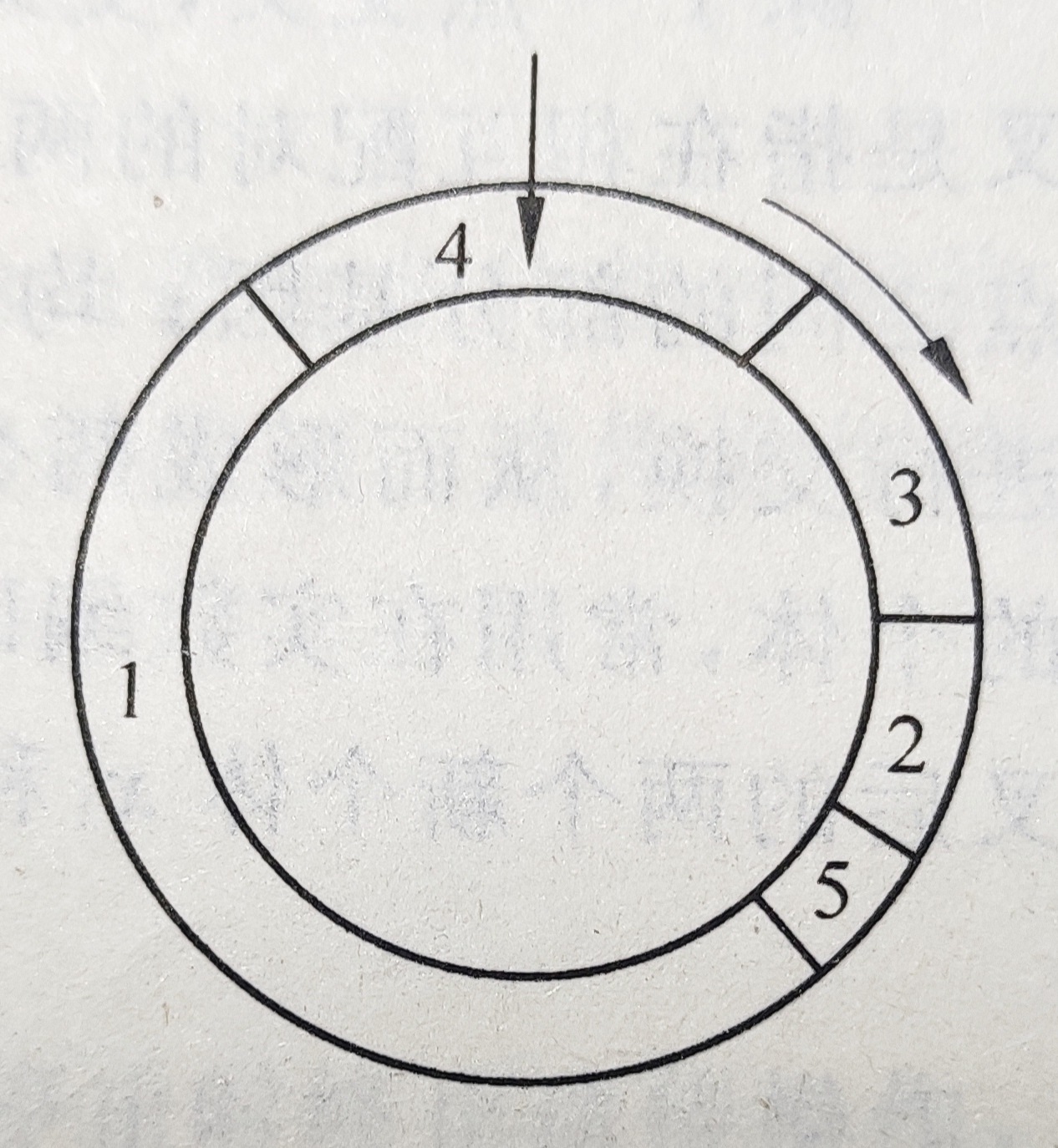• 选择操作是从旧的群体中选出优秀者，但并不生成新的个体

• 遗传操作之交叉猪爸爸和猪妈妈有了孩子：利用来自不同染色体的基因通过交换和重组来产生新一代染色体，从而产生下一代新的个体。通过交叉操作，遗传算法的搜索能力得以大大提高

• 操作过程：在当前群体中任选取两个个体，按给定的交叉概率 P c > r a n d o m [ 0 , 1 ] P_c>random[0,1] 在染色体的基因链上选取交叉位置，将这两个个体从该位置起的末尾部分基因互换得到两个新的染色体
• 交叉操作的方式：
• 一点交叉：在相互配对的两个个体基因编码串中随机设置一个交叉点，并交换交叉点之后的尾部基因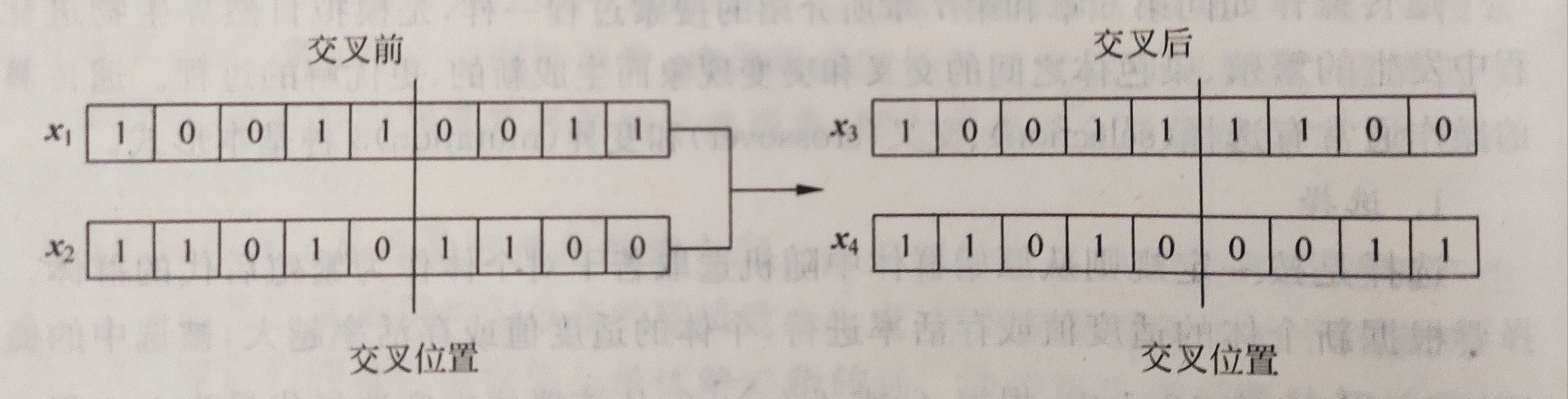• 两点交叉：在相互配对的两个个体基因编码串中随机设置两个交叉点，并交换交叉点之间的部分基因
• 一致（均匀）交叉：两个相互配对的个体的每一位基因都以相同的概率进行交换，从而形成两个新的个体
• 算术交叉：由两个个体的线性组合二产生出新的个体，常用在实数编码的遗传算法中
• 设对 x 1 x_1 x 2 x_2 两个个体进行算术交叉，则交叉后的新个体为 x 1 ′ = α x 1 + ( 1 − α ) x 2 x_1'=\alpha x_1+(1-\alpha )x_2
x 2 ′ = ( 1 − α ) x 1 + α x 2 x_2'=(1-\alpha ) x_1+\alpha x_2
式中， α ∈ ( 0 , 1 ) \alpha \in (0,1) 为一随机数
• 交叉操作是产生新个体的主要方法之一，因此交叉概率 P c P_c 应取较大值。但 P c P_c 取过大值可能会破坏群体中的优良模式，对进化计算产生不利的影响。若 P c P_c 取值较小，则产生新个体的速度较慢，算法效率低
• 遗传算法交叉操作概率 P c P_c 的取值范围一般为0.59~0.99

• 遗传操作之变异猪宝宝变异了，有的会飞了，有的天生站不起来：变异增加了遗传算法找到接近最优解的能力，可以维持群体的多样性，防止出现早熟

• 变异是按给定的变异概率 P m > r a n d o m [ 0 , 1 ] P_m>random[0,1] 改变某个体的某一基因值，以生成一个新的个体
• 变异操作方式:
• 在二进制编码中，就是将变异位置处的基因由0变为1，或由0变为1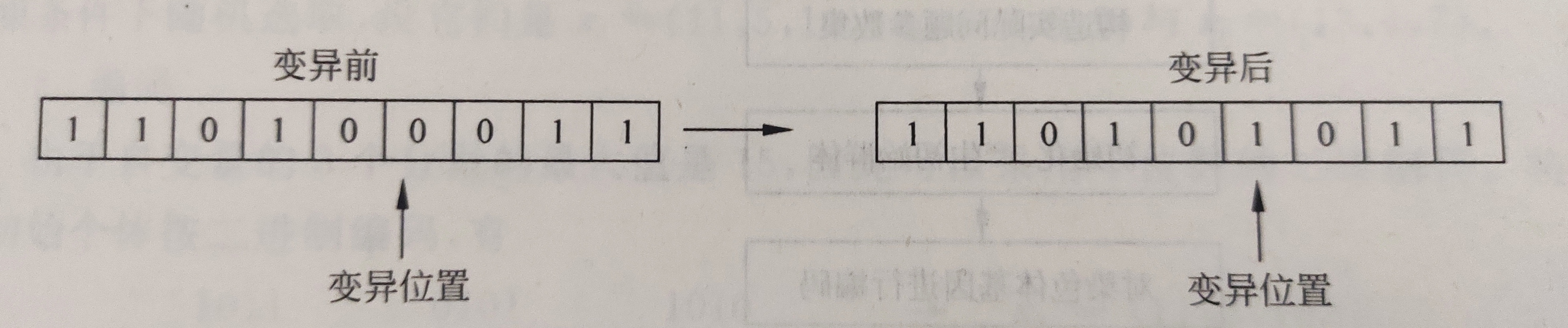• 对于实数编码的变异操作可按下式执行 x ′ = x + 2 ( α − 0.5 ) x m a x x'=x+2(\alpha -0.5)x_{max} 式中， α ∈ ( 0 , 1 ) \alpha \in (0,1) 为一随机数，x为变异前个体， x m a x x_{max} 是x在变异操作中的最大可能改变值，x’是变异后个体
• 变异概率 P m P_m 不宜选取过大，过大可能会把群体中较好的个体变异掉，一般取0.0001~0.1，如果变异概率大于0.5，遗传算法就退化为随机搜索法

• 终止这个猪种群繁衍到终止的时候的现状

1. 根据终止进化代数N，一般取值范围为100~500
2. 根据适度值的最小偏差满足要求的偏差范围
3. 根据适度值的变化趋势，当适度值逐渐趋于缓和或者停止时终止遗传操作，即群体中的最优个体在连续若干代没有改进或平均适度值在连续若干代基本没有改进后即可停止

• 遗传算法流程图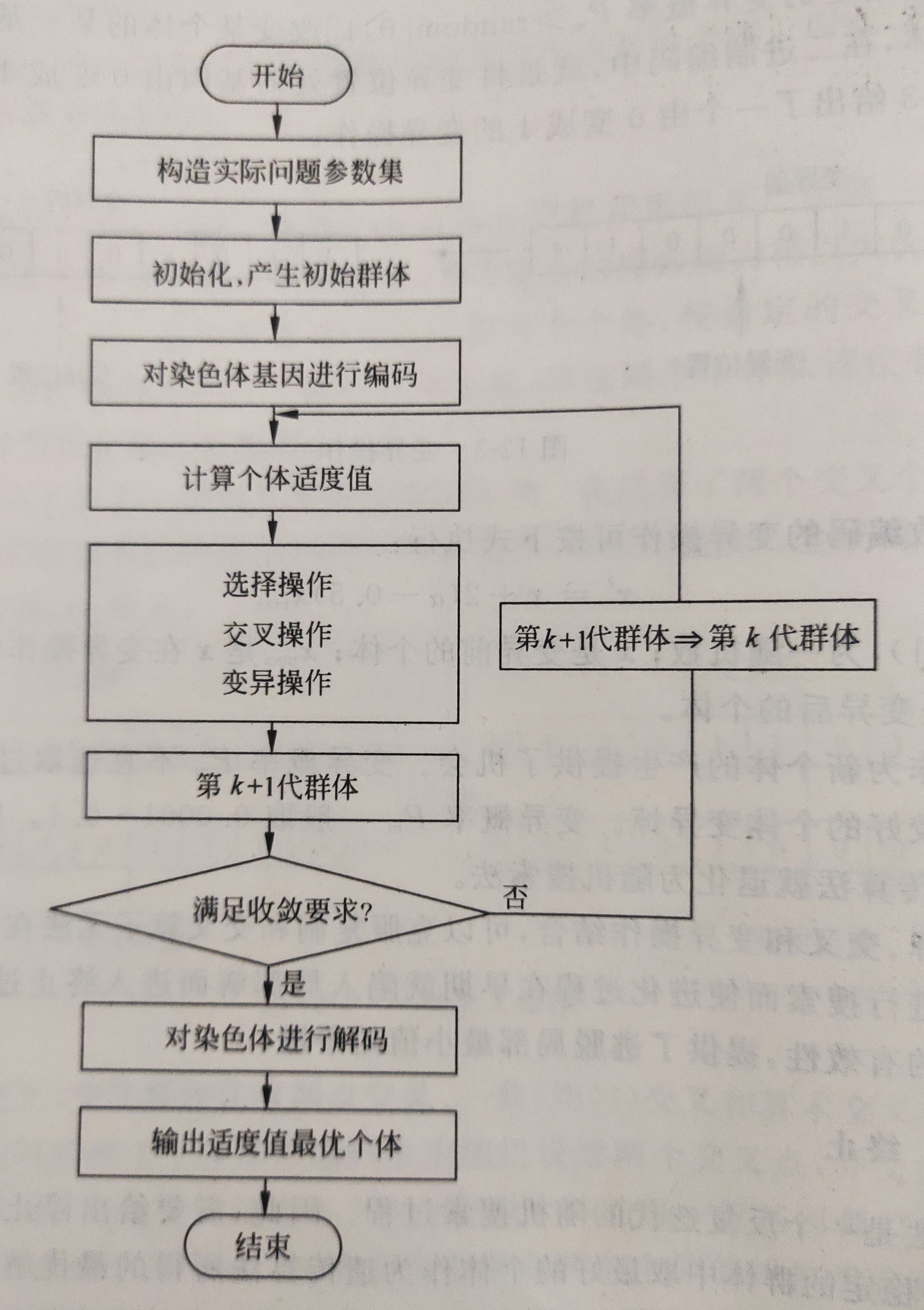实例：

• 求解函数 y = 10 s i n ( 5 x ) + 7 ∗ ∣ x − 5 ∣ + 10 y=10sin(5x)+7*|x-5|+10 在定义域（0,10）上的最大值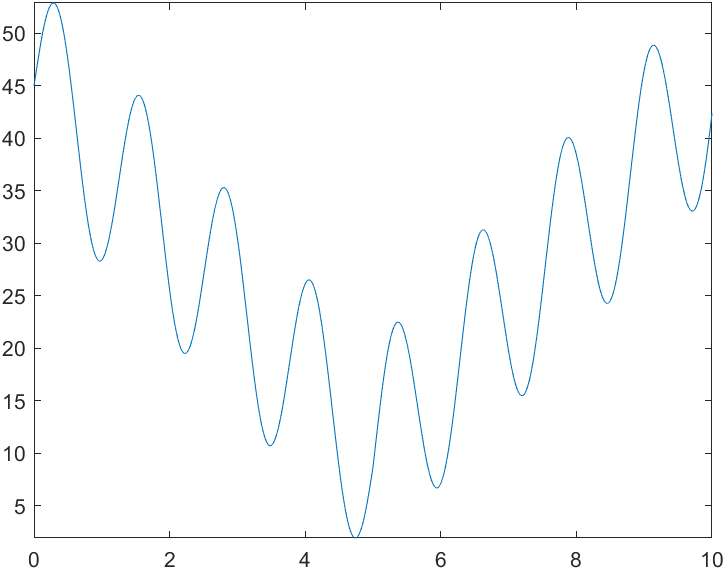1. 主函数

function main()
clear;
clc;
%种群大小
popsize=100;
%二进制编码长度
chromlength=10;
%交叉概率
pc=0.6;
%变异概率
pm=0.001;
%初始种群
pop = initpop(popsize,chromlength);
%迭代次数
time=300;

x=ones(time,1);
fitvalue1=ones(time,1);

for i=1:time
%计算适应度值（函数值）
objvalue=cal_objvalue(pop);
fitvalue=objvalue;
%选择操作
newpop=selection(pop,fitvalue);
%交叉操作
newpop=crossover(newpop,pc);
%变异操作
newpop=mutation(newpop,pm);
%更新种群
pop=newpop;

[bestindividual,bestfit]=best(pop,fitvalue);
x(i)=binary2decimal(bestindividual);
fitvalue1(i)=bestfit;
end
%寻找最优解
x1=binary2decimal(newpop);
y1=cal_objvalue(newpop);
%显示最终种群进化后的优解
figure;
fplot(@(x)10*sin(5*x)+7*abs(x-5)+10,[0 10]);
hold on
plot(x1,y1,'*');
title(['迭代次数为' num2str(time)]);
%显示每一代的最优解的迭代过程
figure;
y=1:time;
plot(y,x,'.');
figure;
plot(y,fitvalue1,'.');

2. 初始种群大小

%初始化种群大小
%输入变量：
%popsize：种群大小
%chromlength：染色体长度->转化的二进制长度
%输出变量：
%pop：种群
function pop=initpop(popsize,chromlength)
pop=round(rand(popsize,chromlength));

3. 二进制转换为十进制函数（注意二进制是用矩阵表示的）

%二进制转化为十进制函数
%输入变量：
%二进制种群
%输出变量：
%十进制数值
function pop2=binary2decimal(pop)
[px,py]=size(pop);
for i=1:py
pop1(:,i)=2.^(py-i).*pop(:,i);
end
temp=sum(pop1,2);
%精度问题，题目定义域长度为10，而10位二进制编码可以表示0~1023
pop2=temp/1023*10;

4. 适应度函数

%适应度函数
%输入变量：二进制数值
%输出变量：目标函数值
function [objvalue]=cal_objvalue(pop)
x=binary2decimal(pop);
objvalue=10*sin(5*x)+7*abs(x-5)+10;

5. 选择函数

%如何选择新的个体
%输入变量：pop二进制种群，fitvalue：适应度值
%输出变量：newpop选择以后的二进制种群
function [newpop]=selection(pop,fitvalue)
%构造轮盘
[px,py]=size(pop);
totalfit=sum(fitvalue);
p_fitvalue=fitvalue/totalfit;
p_fitvalue=cumsum(p_fitvalue);
ms=sort(rand(px,1));
fitin=1;
newin=1;
while newin<=px
if(ms(newin)<p_fitvalue(fitin))
newpop(newin,:)=pop(fitin,:);
newin=newin+1;
else
fitin=fitin+1;
end
end

6. 交叉函数

%交叉变换
%输入变量：pop:二进制父代种群数，pc：交叉概率
%输出变量：newpop：交叉后的种群数
function [newpop]=crossover(pop,pc)
[px,py]=size(pop);
newpop=ones(size(pop));
for i=1:2:px-1
if(rand<pc)
cpoint=round(rand*py);
newpop(i,:)=[pop(i,1:cpoint),pop(i+1,cpoint+1:py)];
newpop(i+1,:)=[pop(i+1,1:cpoint),pop(i,cpoint+1:py)];
else
newpop(i,:)=pop(i,:);
newpop(i+1,:)=pop(i+1,:);
end
end

7. 变异函数

%变异
%输入变量：pop：二进制种群，pm：变异概率
%输出变量：newpop变异后的种群
function [newpop]=mutation(pop,pm)
[px,py]=size(pop);
newpop=ones(size(pop));
for i=1:px
newpop(i,:)=pop(i,:);
if(rand<pm)
mpoint=round(rand*py);
if mpoint<=0
mpoint=1;
end
if newpop(i,mpoint)==0
newpop(i,mpoint)=1;
else
newpop(i,mpoint)=0;
end
end
end

8. 取最优值函数

%求最优适应度函数
%输入变量：pop：种族，fitvalue：种群适应度
%输出变量：bestindividual：最佳个体，bestvalue：最佳适应度值
function [bestindividual,bestfit]=best(pop,fitvalue)
[px,py]=size(pop);
bestindividual=pop(1,:);
bestfit=fitvalue(1);
for i=2:px
if bestfit<fitvalue(i)
bestindividual=pop(i,:);
bestfit=fitvalue(i);
end
end

• 执行结果
• 第一次执行，迭代300次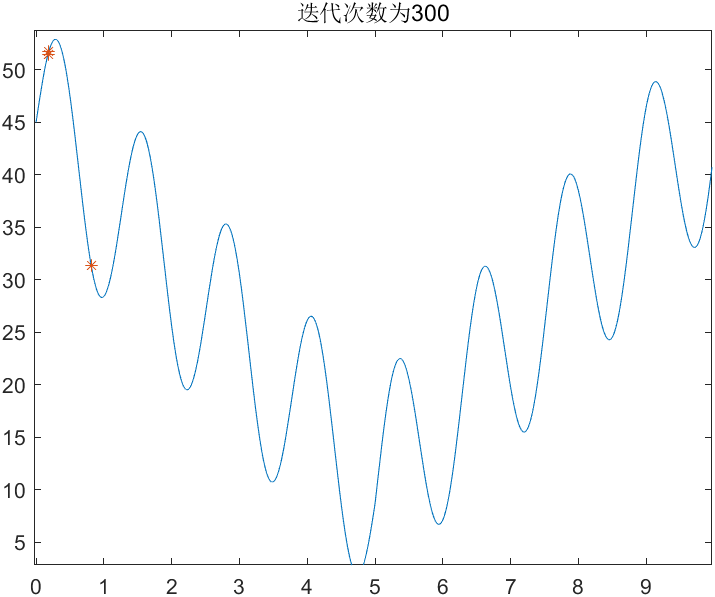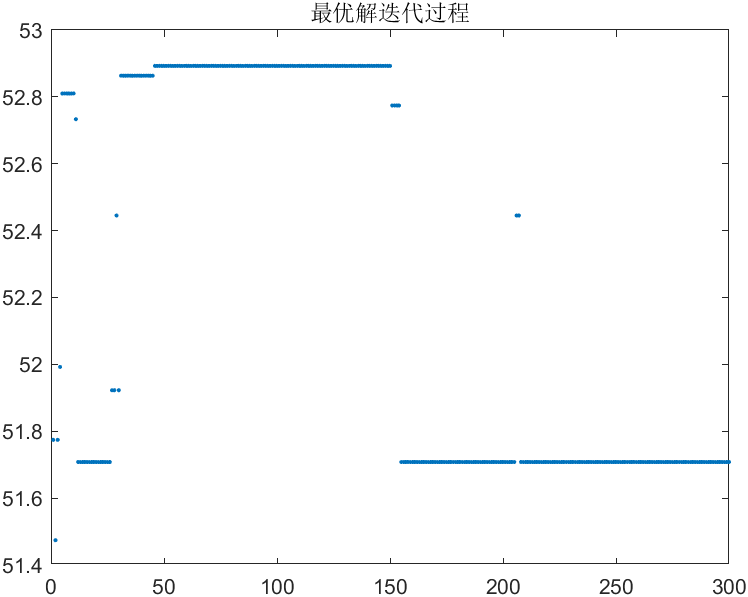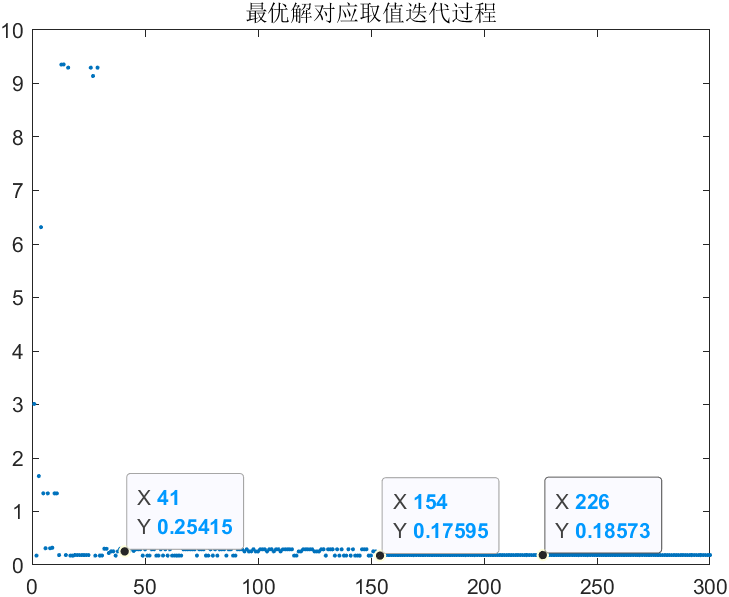纵坐标对应X取值

• 第二次执行，迭代300次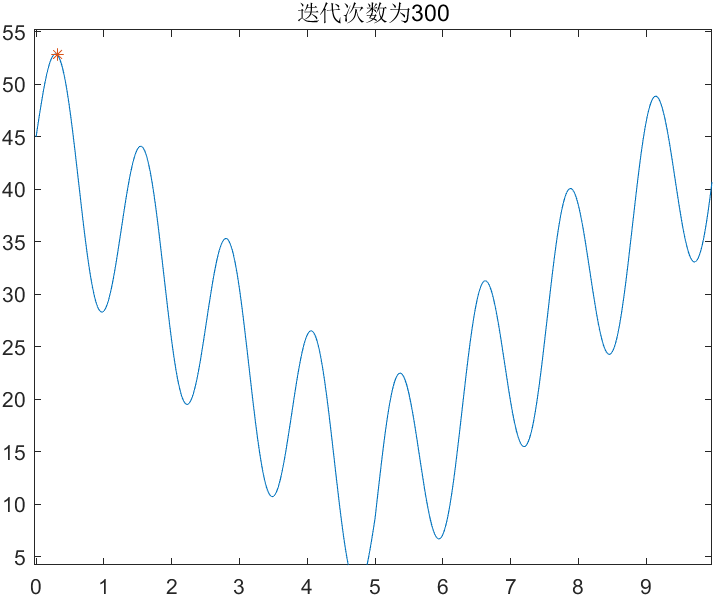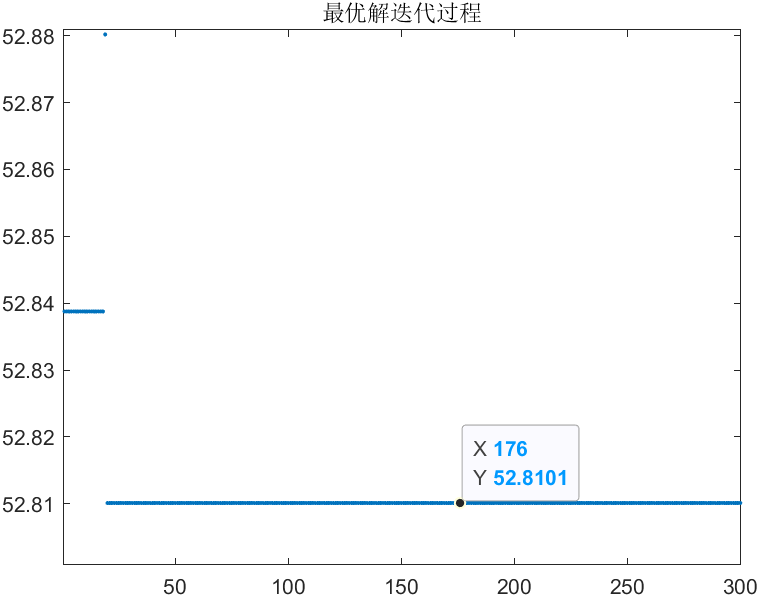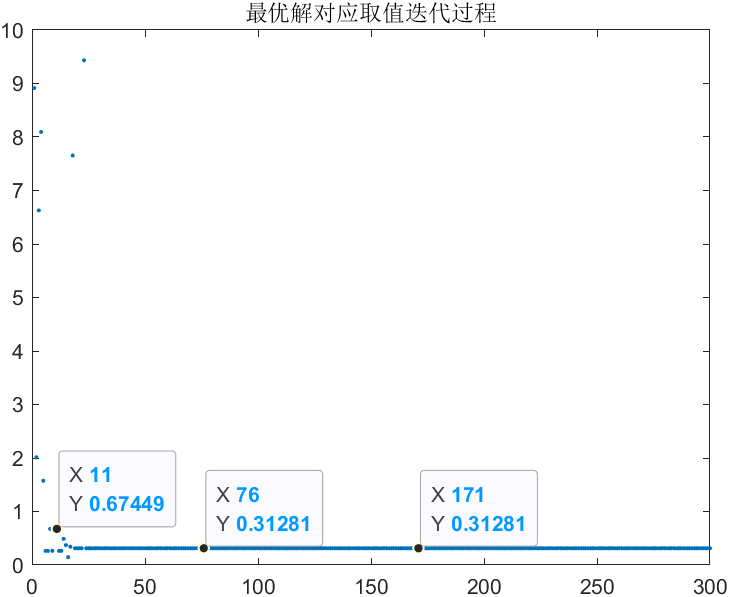纵坐标对应X取值

• 第三次执行，迭代50次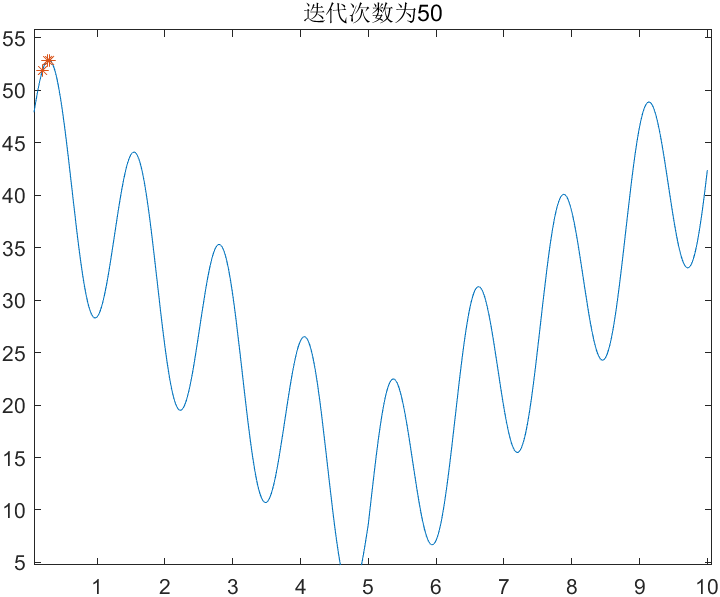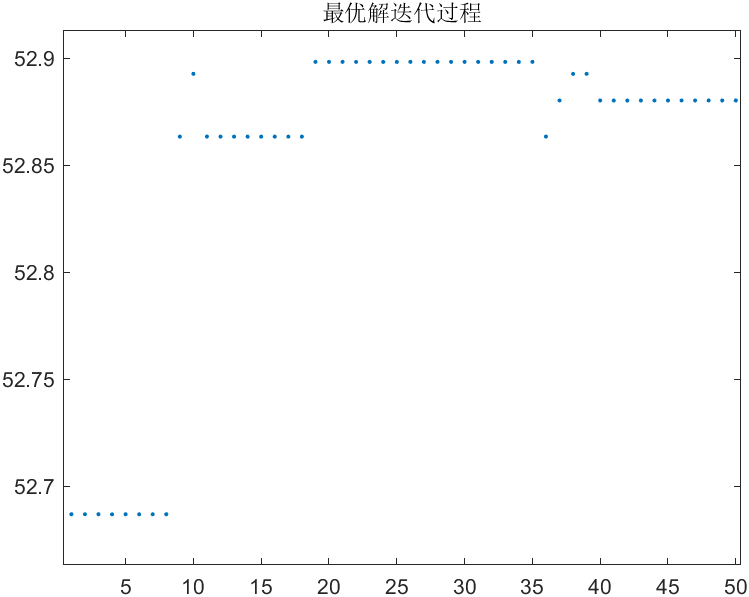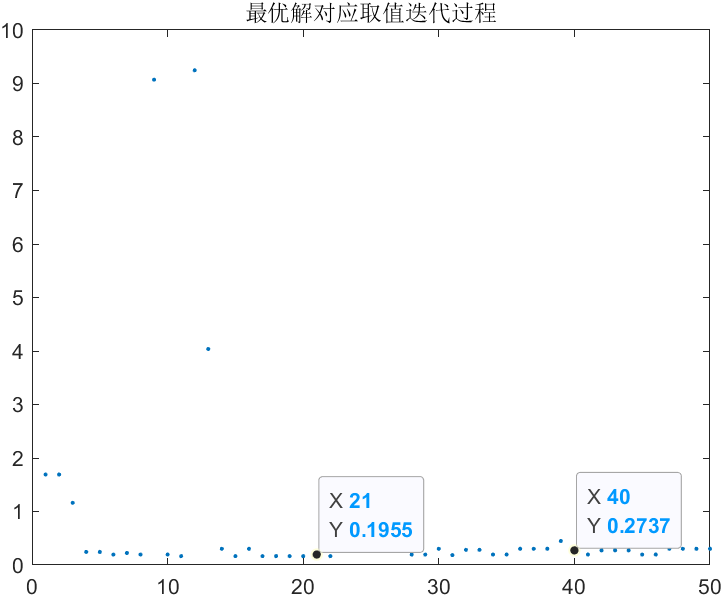纵坐标对应X取值

• 第四次执行，迭代10次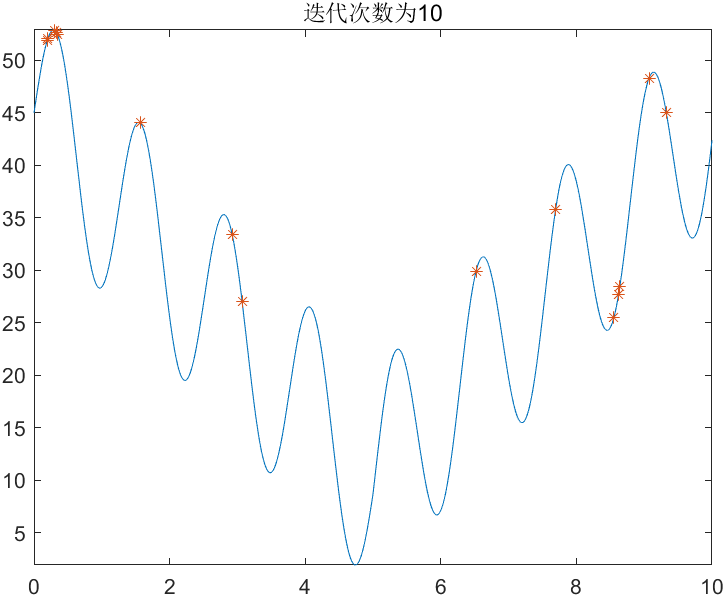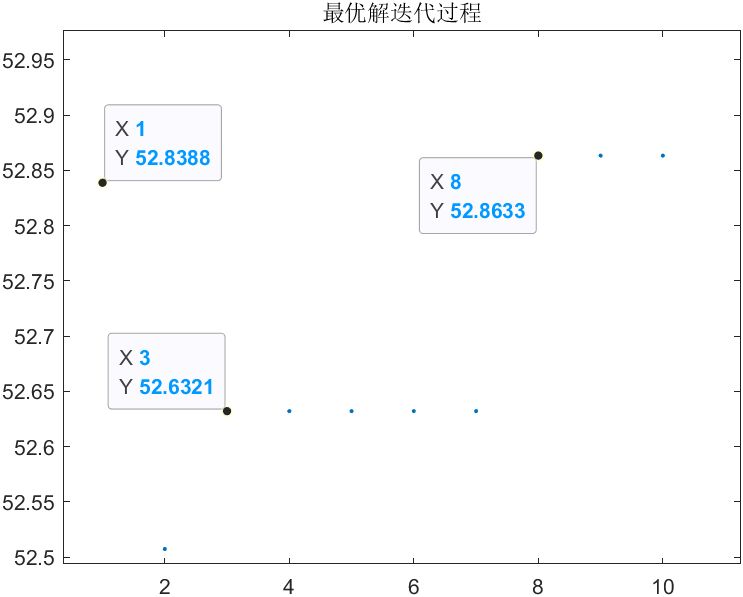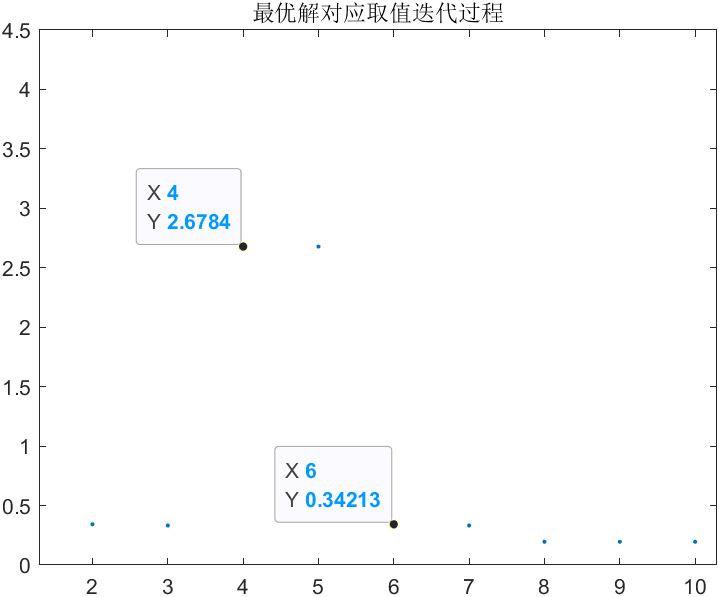纵坐标对应X取值

物竞天择，适者生存

展开全文算法 机器学习 matlab
• 回顾一下数据拟合过程，我们有一组数据，并且知道一般表达式，以及表达式中...接下来，我们通过一个简单的例子，来介绍利用 MATLAB 自带的遗传算法工具箱 (ga) 来进行数据拟合。遗传算法数据拟合创建一组数据，并...

回顾一下数据拟合过程，我们有一组数据，并且知道一般表达式，以及表达式中参数的粗略值，也就是拟合参数的初始值，最后一般都能拟合出精确的参数值。

但是，如果表达式中参数个数较多，各个参数的粗略值估计不准，用一般的方法很容易找到一个局域最优解，而我们需要的是全局最优解。

接下来，我们通过一个简单的例子，来介绍利用 MATLAB 自带的遗传算法工具箱 (ga) 来进行数据拟合。

遗传算法数据拟合

创建一组数据，并加上随机噪声，其代码和图像如下。

x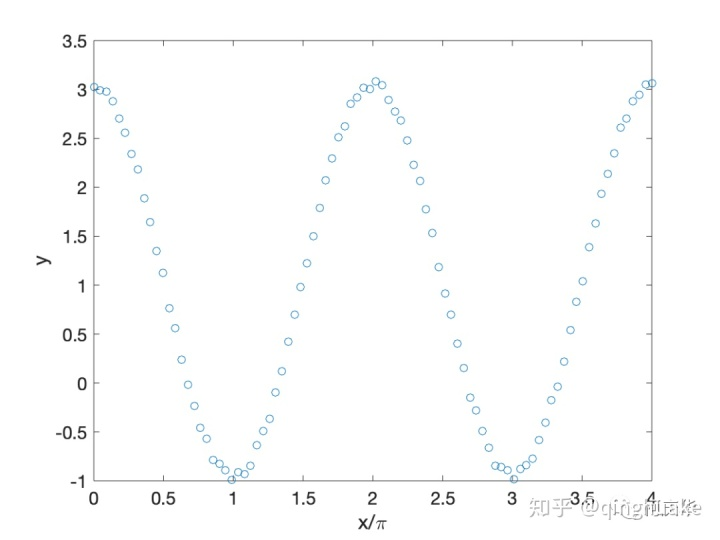数据点满足的一般表达式写成为了拟合出参数值，写出最重要的适应度函数

function

设置各个参数的大致范围

LB

用 ga 函数拟合

ObjectiveFunction

最后得到结果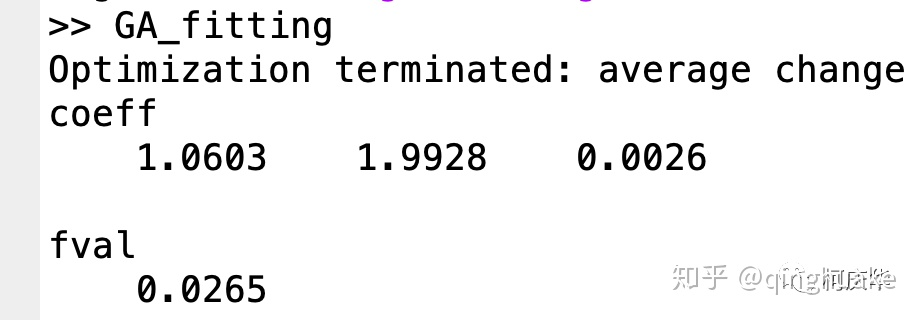得到的结果和其表达式 () 中准确的参数 () 还是挺相近的，偏差 (fval) 来自于加上的噪声。将拟合曲线和数据点画在同一张图中。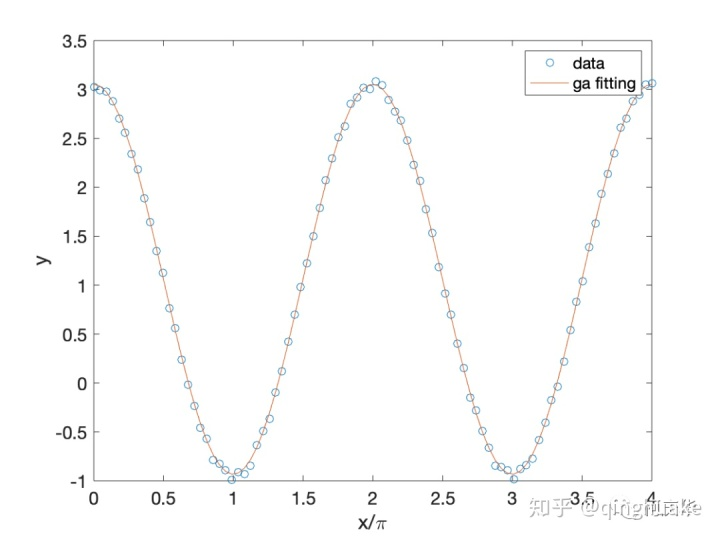用 ga 拟合的优点

对表达式参数的初值要求不高，适合多参数的拟合过程，最重要的是，不用自己写遗传算法真好。

在我的公众号后台回复 GAfit 即可获得源码。

展开全文遗传算法matlab
• 简单遗传算法的Matlab代码实现

千次阅读 多人点赞 2019-04-18 17:17:07
1、遗传算法介绍 遗传算法，模拟达尔文进化论的自然选择和遗产学机理的生物进化构成的计算模型，一种不断选择优良个体的算法。谈到遗传，想想自然界动物遗传是怎么来的，自然主要过程包括染色体的选择，交叉，变异...

1、遗传算法介绍

遗传算法，模拟达尔文进化论的自然选择和遗产学机理的生物进化构成的计算模型，一种不断选择优良个体的算法。谈到遗传，想想自然界动物遗传是怎么来的，自然主要过程包括染色体的选择，交叉，变异（不明白这个的可以去看看生物学），这些操作后，保证了以后的个基本上是最优的，那么以后再继续这样下去，就可以一直最优了。

2、解决的问题

先说说自己要解决的问题吧，遗传算法很有名，自然能解决的问题很多了，在原理上不变的情况下，只要改变模型的应用环境和形式，基本上都可以。但是遗传算法主要还是解决优化类问题，尤其是那种不能直接解出来的很复杂的问题，而实际情况通常也是这样的。

本部分主要为了了解遗传算法的应用，选择一个复杂的二维函数来进行遗传算法优化，函数显示为y=10*sin(5*x)+7*abs(x-5)+10,这个函数图像为：怎么样，还是有一点复杂的吧，当然你还可以任意假设和编写，只要符合就可以。那么现在问你要你一下求出最大值你能求出来吗？（这个貌似可以，很容易写出来----如果再复杂一点估计就不行了）这类问题如果用遗传算法或者其他优化方法九很简单了，为什么呢？说白了，其实就是计算机太笨了同时计算速度又超快，举个例子吧，我把x等分成100万份，再一下子都带值进去算，求出对应的100万个y的值，再比较他们的大小找到最大值不就可以了吗，很笨吧，人算是不可能的，但是计算机可以。而遗传算法也是很笨的一个个搜索，只不过加了一点什么了，就是人为的给它算的方向和策略，让它有目的的算，这也就是算法了。

3、如何开始？

不明白遗传算法的会问怎么开始呢？恩，其实每个算法都有自己的开始方式，遗传算法也是，首先是选择个体了。我们知道一个种群中可能只有一个个体吗？不可能吧，肯定很多人才对，这样相互结合的机会才多，产生的后代才会多种多样，才会有更好的优良基因，有利于种群的发展。那么算法也是如此，当然个体多少是个问题，一般来说20-100之间我觉得差不多了。那么个体究竟是什么呢？在我们这个问题中自然就是x值了。其他情况下，个体就是所求问题的变量，这里我们假设个体数选100个，也就是开始选100个不同的x值，不明白的话就假设是100个猴子吧。好了，现在有了100个猴子组成的一个种群，那么这个种群应该怎么发展才能越来越好？说到这，我们想想，如何定义这个越来越好呢？这个应该有一个评价指标吧。在我们的这个问题中，好像是对应的y值越大越好是吧。我们甚至可以给他们排个名来决定哪些好哪些不好。我们把这个叫做对于个体的适应度，这应该算是算法的后半部分才对。

4、编码

首先明白什么是编码？为什么要编码？如何编码？

好，什么是编码？其实编码就是把自变量（x）换一下形式而已，在这个形式下，更容易操作其他过程（比如交叉，变异什么的）而已。举个例子吧，加入我们取x=1, 2, 3，我们可以把x 编码成x=a, b, c,我就说123对应就是abc，为什么要这样做呢？比如问题里面你能够获取的都是abc的组合之类的，那么这样编码以后，你就可以再返回去成123来操作了。一般的编码都是些什么呢？二进制编码，自然数编码，矩阵编码。。等等，很多，不详细写了。而用的最多的可以说是二进制编码，感觉这和人体DNA，基因的排列很相似。想想DNA怎么排的？不就是在两条长链上一对一排的吗？那么什么是二进制编码？很简单，就是1,0,1,0对应的来回组合排列而已。比如：1100100010, 0011001001等等，这些都是位数长度为10的二进制编码。再想想1在计算机的二进制形式是什么？如果八位来表示的话，是不是就是0000 0001；8是不是就是0000 1000；以此类推，那么我们这里也是这样，把对应的x值换算成这种编码形式，我们这里可以看到x的范围是0-5吧，如何按照计算机这样的方式是不是到0000 0101这里就完事了？想想这样多短，前面五位都没有用上多浪费呀，那么要想都用上怎么办呢？也很简单，我们把0000 0001不认为是1不就可以了吗？因为1111 1111是255，那么如果说每一份为1/255的话，那么0000 0001不就是1/255了吗？这个时候1怎样表示了？不就是1111 1111吗？好了我们把范围扩大一些吧，每一份不是1/255,而是1/255*5,那么这个时候最大值是多少？是不是5，恩，这样x编码的范围不就在0-5之间了吗？这里就又有问题了，想想这样做的话，x的最小精度是多少？就是1/255*5.虽然很小，但是在0-1/255*5之间的数你能不能取到？无论如何都取不到吧。那么就又来一个问题，怎样去扩大这个精度呢？如果要保持0-5不变的话，只能增加位数了，把9位编码编程10位，20位，100位，哇，够大了吧，变成100个0,1组合，很恐怖吧，事实上，究竟是多少要视情况而定，一般20位左右感觉就可以了，虽然说越大越好，但是太大了消耗内存，速度慢了，不值。本题中，我们设置它为一个变量，先暂时取为10来实验，好了，如果还不明白为什么要编码看下面的吧。知道了交叉与变异，你就知道了。

5、关于交叉与变异

先说变异，什么是变异？简单，基因发生突变就叫变异，有了编码的概念，那就在编码基础上来说变异。首先就讲最简单的变异，但个点的变异。现在以10位长的编码来说，比如把x=3编码一下，随便假设为11000 10010吧，好了，在变异操作时，假设第5位变异了（说一下变异就是一位或者多位1或0变成0或1，也只能0,1之间变，没办法啊），那么这个时候变成什么了？是不是11001 10010再反编码回去成x是多少呢？那肯定不是3了，变了呀，是多少是肯定可以反算回去的，这里懒得算了，就假设为3.213吧，发没发现，这样一来，x是不是变了？既然变了就好啊，带到原函数（适应度函数）里面比较这两个x值对应的哪个y值大一写，如果后面变异后的大一些是不是就是说产生了好的变异啊，就可以在下一次个体选择的时候选择它了。那么想想很多x来一起变异会怎么样呢？肯定会生成很多很多的解吧，反复这么做会怎么样呢？只要每次都保留最优解的话，我来个循环100万次，也总能找到解吧，当然这么多次得花多久，也不太合适，这还只是一个点位在进行变异，若果每次我让多个点位变异呢？哇，又不可思议了，变化更大了吧。当然，变异不止如此，更多的去看专业的论文吧。知道了变异是干什么的，剩下的都好说了，好了，这还是变异，想想自然界遗传中除了变异还有什么？交叉吧，那么交叉又是什么？

学过生物的都知道，动物交配时，部分染色体干什么了？是不是交叉了？就是把相应部分的基因交换了，你的给我，我的给你，很有爱吧。再以编码为例，比如现在随便从100个x值中选取两个吧，鸡舍正好选中了x=3和4，对应的编码假设是11001 10101 和00101 01011，那么怎么交叉呢？我们知道每次交叉的染色体通常是一块一块的，恩，这里在算法设计上也来一块一块的吧。比如说就把位置在2,3,4号的编码给整体交叉了，那么x=3对应的位置是100吧，x=4对应的位置是010吧，好，交换以后x=3对应的位置就变成了010，x=4对应的位置就变成100，加回去就变成什么了？x=3是不是就是10101 10101，x=4是不是就是01001 01011了。而现在，把他们再反编码回去还是x=3和x=4吗？显然又不是了吧（当然也有小概率是一样的吧，很小）。那是什么？不想算，还是假设吧，假设为3.234和4.358吧，好了新的个体是不是又来了？恩，同理，带到适应度函数里面去吧，再取优秀个体，完事。同样，有些专门研究这种算法的开发出来各种各样的交叉方式，什么一个个体的钱3个与后一个个体的后三个交叉，中间几位来交叉等等，总之就是生产新个体。而这样做的目的在哪呢？无非是三个字，随机性，充分保证生产新个体具有随机性，你说你的x=3变异后为3.2,3.2什么的距离3那么近，在一些存在局部最优解的问题上就永远跳不出局部最优解，相反，你的x=1一下子变异成了x=5，哇，好大的变化，一下从这头到了那头，这对于算法的广阔搜索能力来说是非常好的。

讲完了这部分，现在知道了为什么要编码了吧？如果你不编码，你说你想要你的x=3怎么去变异？怎么去交叉？当然也不是没有方法，比如你生成一个小的随机数加到x=3上，但是你想想这两种方法哪一个更具有随机性、普遍性？显然的。而更多的时候交叉预编译是在一起操作的，先交叉，再变异是普遍遗传算法的操作步骤。

6、关于选择的问题

说完了上面的部分，再说说选择吧，选择是什么？就是优胜劣汰。好的留下来，差的走人，在自然界中直接gg了是吧。不停地选择是的种群一直吵着较好的方向行走。

对应到本问题来说，遗传算法的选择是什么样子？在前面说到，每次交叉或者变异是不是产生了新的个体？如果这些个体都保留下来的话，种群是要爆炸的，第一次循环可能有100个x，第二次循环就200个个体，再来那么10万次，哇哦，多少了，好多。显然不可能吧。而且在算法里面，我们还规定的是每次循环都必须保证都是100个个体。那么必须在200个个体中剔除100个吧。好了，问题来了，如何剔除呢？有人说很简单，排名吧，取前100号x不就可以了吗？排名这个东西真的好吗？我就不信，凭什么差一点的不能选上，搞不好在下一次变异中一下子冲到了第一呢？这个问题在选择上也有一些对应的规则，最通用的就是轮盘赌法，简单来说就是一种概率选择法（当然还有许多其他的方法，感兴趣的自己搜相关的文献吧，我也没用过）。什么是轮盘赌法呢？就是把对应所有y值（适应度函数值）加起来，在用各自的y值去除以这个sum值，这样是不是谁的概率大谁的概率小就很清楚了？然后再随机生成一个0-1的概率值p，谁在p的范围里面是不是就选择谁，比如说x=3时在100个x中y的值最大，那么选择它的概率是不是就最大，比如说是0.1（0.1小吗？不小了好吧，想想其他的会是什么，都比0.1小，那么从概率上讲，选100次的话，是不是就有10次宣导x=3，其他的都不足10次是吧，那么在下一次100个种群个体中就有10个x=3了，再来一回可能就有20个x=3的个体了。再就是30个，最后就只剩下100个x=3的个体了，它自己在哪里交叉变异已经没有意义了，如果到了这个时候就意味着这个算法可以结束了）。再详细点，如下图所示吧：现在要在下面三个大类中选取100个x个体，轮盘赌转100次以后，是不是个艺术落在s3中的个体多一些，选择的原理就是这样，再不明白直接后面的程序吧。7、还差点什么呢

至此，感觉也差不多了吧，选择完后在重复上述步骤交叉，变异等等，那么什么时候是个头了？很简单，办法就是迭代次数，迭代10次看一下结果，20次，看一下结果，30次，40次，100次，当次数达到一定程度以后，优秀的个体越来越多，大都集中在最优解附近，即使变异或者交叉了也是在这个最优解附近，没有影响的。在下一次选择就又变回来了，那么至此就真的结束了。比如说先来结果吧，该问题按我的思路做完后，迭代100次变成什么样子了？看图：下面看代码：

（1）首先看主函数

1. function main()
2. clear;
3. clc;
4. %种群大小
5. popsize= 100;
6. %二进制编码长度
7. chromlength= 10;
8. %交叉概率
9. pc = 0.6;
10. %变异概率
11. pm = 0.001;
12. %初始种群
13. pop = initpop(popsize,chromlength);
14. for i = 1: 100
15. %计算适应度值（函数值）
16. objvalue = cal_objvalue(pop);
17. fitvalue = objvalue;
18. %选择操作
19. newpop = selection(pop,fitvalue);
20. %交叉操作
21. newpop = crossover(newpop,pc);
22. %变异操作
23. newpop = mutation(newpop,pm);
24. %更新种群
25. pop = newpop;
26. %寻找最优解
27. [bestindividual,bestfit] = best(pop,fitvalue);
28. x2 = binary2decimal(bestindividual);
29. x1 = binary2decimal(newpop);
30. y1 = cal_objvalue(newpop);
31. if mod(i, 10) == 0
32. figure;
33. fplot( '10*sin(5*x)+7*abs(x-5)+10',[ 0 10]);
34. hold on;
35. plot(x1,y1, '*');
36. title([ '迭代次数为n=' num2str(i)]);
37. %plot(x1,y1, '*');
38. end
39. end
40. fprintf( 'The best X is --->>%5.2f\n',x2);
41. fprintf( 'The best Y is --->>%5.2f\n',bestfit);

（2）下面看二进制种群生成的方法

1. %初始化种群大小
2. %输入变量：
3. %popsize：种群大小
4. %chromlength：染色体长度-->>转化的二进制长度
5. %输出变量：
6. %pop：种群
7. function pop=initpop(popsize,chromlength)
8. pop = round (rand(popsize,chromlength));
9. %rand( 3, 4)生成 34列的 0- 1之间的随机数
10. % rand( 3, 4)
11. %
12. % ans =
13. %
14. % 0.8147 0.9134 0.2785 0.9649
15. % 0.9058 0.6324 0.5469 0.1576
16. % 0.1270 0.0975 0.9575 0.9706
17. %round就是四舍五入
18. % round(rand( 3, 4))=
19. % 1 1 0 1
20. % 1 1 1 0
21. % 0 0 1 1
22. %所以返回的种群就是每行是一个个体，列数是染色体长度

（3）下面看如何把二进制返回对应的十进制

1. %二进制转化成十进制函数
2. %输入变量：
3. %二进制种群
4. %输出变量
5. %十进制数值
6. function pop2 = binary2decimal(pop)
7. [ px, py]= size (pop);
8. for i = 1:py
9. pop1(:,i) = 2.^(py-i).*pop(:,i);
10. end
11. %sum(., 2)对行求和，得到列向量
12. temp = sum(pop1, 2);
13. pop2 = temp* 10/ 1023;

输入的是100组0,1编码的二进制，输出的是x值，开始取一下种群大小，size（pop）,显然这里py是10了，借着对每一位求和，就是pop1（:,i）=2.^(py-i).*pop(:,i);这里省略用了冒号，，什么依稀呢？就是对所有行都有这个操作，冒号意思就是胸1到100了，那么就其中一个个体来说吧，假设为11001 10010，那么先进性这么一个操作就是什么呢？是不是就是对应的为0或1乘以2的对应次幂，如果1就是管用，是0就不管用。那么这个值就是(2^0)*1+(2^1)*1+0+0+(2^4)*1+....这样就算出了一个值，因为是10位编码，所以这个数是结余0-2^9即0-1023.那么最大为多少？1023吧。temp = sum(pop1,2)是对行求和吧，2表示行，1表示列，最后一行是吧它转化为100组0-10之间的数值了。

（4）下面看计算适应度函数：

1. %计算函数目标值
2. %输入变量：二进制数值
3. %输出变量：目标函数值
4. function [objvalue] = cal_objvalue(pop)
5. x = binary2decimal (pop);
6. %转化二进制数为x变量的变化域范围的数值
7. objvalue= 10*sin( 5*x)+ 7*abs(x- 5)+ 10;

（5）如何选择新的个体

上面所有个体的函数值都计算出来了，存在objvalue中，此时它是不是也是100组y值啊，恩，那么对于现有的随机生成的100组x，怎么来再选择100组新的更好的x呢？这里我们把选择放在了交叉与变异之间了，都可以，如何选择，就要构造概率的那个轮盘了，谁的概率大，是不是选择的个体就会多一些？也就是现在的选择就是100中100个，最后出现的就够就是以前的100个中最优的x有一个的话，选择完后，可能就变成5个这个x了，多余的4个是不是相当于顶替了以前的不好的4个x值，这样才能达到x总数100不变啊。

1. %如何选择新的个体
2. %输入变量：pop二进制种群，fitvalue：适应度值
3. %输出变量：newpop选择以后的二进制种群
4. function [newpop] = selection(pop,fitvalue)
5. %构造轮盘
6. [px,py] = size(pop);
7. totalfit = sum(fitvalue);
8. p_fitvalue = fitvalue/totalfit;
9. p_fitvalue = cumsum(p_fitvalue);%概率求和排序
10. ms = sort(rand(px, 1));%从小到大排列
11. fitin = 1;
12. newin = 1;
13. while newin<=px
14. if(ms(newin))<p_fitvalue(fitin)
15. newpop(newin,:)=pop(fitin,:);
16. newin = newin+ 1;
17. else
18. fitin=fitin+ 1;
19. end
20. end

（6）怎么交叉

1. %交叉变换
2. %输入变量：pop：二进制的父代种群数，pc：交叉的概率
3. %输出变量：newpop：交叉后的种群数
4. function [newpop] = crossover(pop,pc)
5. [ px, py] = size (pop);
6. newpop = ones(size(pop));
7. for i = 1: 2:px- 1
8. if(rand<pc)
9. cpoint = round(rand*py);
10. newpop(i,:) = [pop(i, 1:cpoint),pop(i+ 1,cpoint+ 1:py)];
11. newpop(i+ 1,:) = [pop(i+ 1, 1:cpoint),pop(i,cpoint+ 1:py)];
12. else
13. newpop(i,:) = pop(i,:);
14. newpop(i+ 1,:) = pop(i+ 1,:);
15. end
16. end

（7）怎么变异

1. %关于编译
2. %函数说明
3. %输入变量：pop：二进制种群，pm：变异概率
4. %输出变量：newpop变异以后的种群
5. function [newpop] = mutation(pop,pm)
6. [ px, py] = size (pop);
7. newpop = ones(size(pop));
8. for i = 1:px
9. if(rand<pm)
10. mpoint = round(rand*py);
11. if mpoint <= 0;
12. mpoint = 1;
13. end
14. newpop(i,:) = pop(i,:);
15. if newpop(i,mpoint) == 0
16. newpop(i,mpoint) = 1;
17. else newpop(i,mpoint) == 1
18. newpop(i,mpoint) = 0;
19. end
20. else newpop(i,:) = pop(i,:);
21. end
22. end

（8）选择最优个体

1. %求最优适应度函数
2. %输入变量：pop:种群，fitvalue:种群适应度
3. %输出变量：bestindividual:最佳个体，bestfit:最佳适应度值
4. function [bestindividual bestfit] = best(pop,fitvalue)
5. [ px, py] = size (pop);
6. bestindividual = pop( 1,:);
7. bestfit = fitvalue( 1);
8. for i = 2:px
9. if fitvalue(i)>bestfit
10. bestindividual = pop(i,:);
11. bestfit = fitvalue(i);
12. end
13. end

到这里遗传算法程序就算是结束了。

看部分图片结果：展开全文遗传算法 Matlab代码
• 路径规划主要是让目标对象在规定范围内的区域内找到一条从起点到终点的无碰撞安全路径。路径规划中有静态路径规划以及动态路径规划，本文所讨论的...本文采用遗传算法和模拟退火混合遗传算法进行求解，得到最优路径。

一、问题描述

路径规划主要是让目标对象在规定范围内的区域内找到一条从起点到终点的无碰撞安全路径。路径规划中有静态路径规划以及动态路径规划，本文所讨论的问题仅针对静态路径规划。具体问题描述如下：
给定起点、终点和障碍物等环境信息，如图1.1所示，利用演化计算方法得到最优轨迹，并分析不同参数选择对结果的影响。本文采用遗传算法和模拟退火混合遗传算法进行求解，得到最优路径。二、遗传算法设计

2.1 算法原理

遗传算法（Genetic Algorithm，GA）是进化计算的一部分，是模拟达尔文的遗传选择和自然淘汰的生物进化过程的计算模型，是一种通过模拟自然进化过程搜索最优解的方法。该算法简单、通用，鲁棒性强，适于并行处理。基本遗传算法流程包括种群初始化、选择、交叉、变异等操作，如图2.1所示。遗传算法的进化过程从完全随机生成的种群开始，随后逐代向适应度更高的种群进化。在进化的每一代中，整个种群的适应度按照一定规则被评价，基于个体的适应度高低从当前种群中随机选择多个个体，通过个体自然选择和基因突变操作而产生新种群，生成的新种群在算法的下一次迭代中成为当前种群。在遗传算法中，需解决的问题的解通常被成为基因个体，它通常以参数列表的形式进行标示，被称作染色体或基因串。其中染色体通常以编码形式体现，即表达为简单的字符串或数字串。

针对本问题，使用遗传算法求解最优路径需要按照特定规则初始化种群，并且根据问题所给的限定条件设计合适的适应度计算方法。下面的内容会详细说明这两个步骤，而算法中的其他操作，如选择、交叉、变异分别采用经典的轮盘赌、单点交叉和随机变异方法，这里不再赘述。

2.2 编码

针对两点间避障路径规划问题，考虑将种群中的每一个个体作为一条路径，个体由一系列坐标点 ( x , y ) (x, y) 组成，所以个体的染色体长度为坐标点数。为了编程方便，这里将个体的坐标分开存储，得到两个种群 P o p x Popx P o p y Popy ，在进化过程中将两个种群看作一个种群，共同优化。
P o p x i = ( x 1 , x 2 , … , x j ) , P o p y i = ( y 1 , y 2 , … , y j ) . Pop{x_i} = ({x_1},{x_2}, \ldots ,{x_j}),Pop{y_i} = ({y_1},{y_2}, \ldots ,{y_j}).

其中 P o p x i Popx_i P o p y i Popy_i 表示种群中的第i个个体， i ∈ [ 1 , M ] i \in \left[ {1,M} \right] x j x_j y j y_j 为个体染色体中的一对编码，表示一个点的横纵坐标， j ∈ [ 1 , N ] j \in \left[ {1,N} \right] M M N N 分别表示种群数量和染色体长度。

2.2 适应度函数

适应度函数要有效反映每一个体与问题的最优个体之间的差距。遗传算法在进化搜索中基本不利用外部信息,仅以适应度函数为依据,利用种群中每个个体的适应度值来进行搜索。本问题的目标是路径总长度为最短，路径总长度的倒数就可以作为适应度函数：
F i t n e s s = 1 ∑ i = 1 M − 1 ( x i + 1 − x i ) 2 + ( y i + 1 − y i ) 2 {\rm{ Fitness }} = \frac{1}{{\sum\limits_{i = 1}^{M - 1} {\sqrt {{{({x_{i + 1}} - {x_i})}^2} + {{({y_{i + 1}} - {y_i})}^2}} } }}

式中， x i x_i y i y_i 是种群中第i个个体的坐标； M M 为种群规模。

为了计算距离，需要判断点和两点连线与障碍物的关系，即判断个体染色体的 x ， y x，y 编码对是否在圆形障碍物内，以及相邻编码对所连成的线段是否与圆形障碍物有交点。若存在点 ( x , y ) (x, y) 不满足上式的条件，则将该个体的适应度置零。
( x − x 0 ) 2 + ( y − y 0 ) 2 > r 2 {(x - {x_0})^2} + {(y - {y_0})^2} > {r^2}
经过上一步的判断，符合条件的个体编码坐标点都在圆形障碍物外，所以这时判断相邻两坐标点组成的线段与障碍物的关系可分为如图2.2所示的三种情况讨论：在分情况讨论之前，还需要计算圆心到直线的距离 d d ，这是为了区别圆心在直线上的特殊情况。计算公式为：
d = ∣ A x 0 + B y 0 + C ∣ A 2 + B 2 d = \frac{{\left| {A{x_0} + B{y_0} + C} \right|}}{{\sqrt {{A^2} + {B^2}} }}

对于第一种情况和第二种情况， d < r d<r 。计算 ∠ O A B \angle OAB ∠ O B A \angle OBA 的余弦函数，若都大于0则该线段与圆相交，不符合条件，将该个体的适应度置零。计算公式为：

cos ⁡ ∠ O A B = A O → ⋅ A B → ∣ A O → ∣ ∣ A B → ∣ > 0 ⇒ A O → ⋅ A B → > 0 \cos \angle OAB = \frac{{\overrightarrow {AO} \cdot \overrightarrow {AB} }}{{\left| {\overrightarrow {AO} } \right|\left| {\overrightarrow {AB} } \right|}} > 0 \Rightarrow \overrightarrow {AO} \cdot \overrightarrow {AB} > 0

对于第三种情况，需要判断BA和BO的距离， d = 0 d=0 。若满足 ∣ B A → ∣ > ∣ B O → ∣ \left| {\overrightarrow {BA} } \right| > \left| {\overrightarrow {BO} } \right| ，则证明该线段穿过圆，不符合条件，将该个体的适应度置零。

具体地，计算适应度函数的算法为：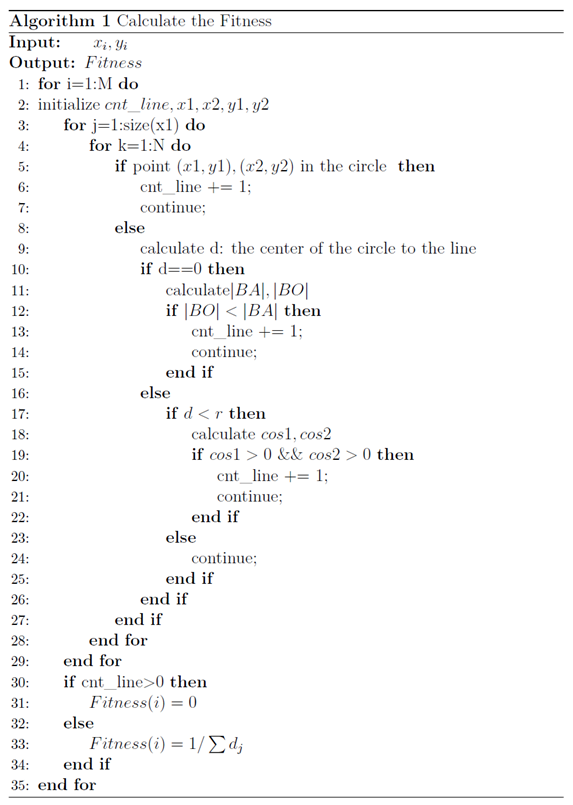2.3 混合遗传算法

为了提高遗传算法运行效率和求解质量，这里引入模拟退火算法的思想，模拟退火(Stimulated Annealing, SA)具有较强的局部寻优能力，并能使搜索过程避免陷入局部最优解[3-4]，采用模拟退火遗传算法（Stimulated Annealing Genetic Algorithm，SAGA）求解路径优化问题。

具体来说，模拟退火遗传算法是对适应度函数的改进，在前期（高温）减少个体间的适应度差异，后期（低温）放大个体间适应度差异，突出优秀个体，完成适应度拉伸的作用，在一定程度上弥补了遗传算法在前期容易早熟、后期容易停滞的缺点。具体的适应度值计算公式如式（6）和（7）所示：
f i = e f i T ∑ i = 1 M e f i T {f_i} = \frac{{{e^{\frac{{{f_i}}}{T}}}}}{{\sum\limits_{i = 1}^M {{e^{\frac{{{f_i}}}{T}}}} }}

T = T 0 ( A g − 1 ) T = {T_0}({A^{g - 1}})
其中， M M 为种群大小， f i f_i 为第 i i 个个体的适应度， g g 为遗传代数， T T 为温度， T 0 T_0 为初始温度， A A 为退火速度（取0.99）。

三、实验结果及分析

本文实验环境为MATLAB2018b，探讨遗传算法的参数设置对结果的影响，比如迭代次数、交叉概率、变异概率，同时比较在相同参数条件下基本遗传算法和混合遗传算法的性能。最后通过可视化结果和路径距离长度来评估算法的准确性与有效性。四、总结

本次作业通过遗传算法编程求解了障碍物路径规划问题，对于基本遗传算法，探讨了算法中迭代次数、交叉概率和变异概率等参数对优化结果的影响。实验结果表明迭代次数不能设置得过小，否则算法还未收敛，反之则会导致计算资源的浪费；交叉概率和变异概率的大小影响种群的多样性，这两个参数过大会导致震荡，无法收敛，反之会导致优化出现停滞。此外，还将模拟退火的思想引入遗传算法，采用模拟退火遗传算法对本问题进行优化。实验结果证明GA算法更适用于该问题的优化求解，这是由于对该问题的个体坐标点排序和SAGA更强局部搜索能力导致无法使搜索过程进入最有希望的搜索区域共同造成的，同时SAGA较多的参数量也对算法寻优调参带来困难。

参考文献

 Holland J H. Genetic algorithms[J]. Scientific american, 1992, 267(1): 66-73.
 王小平, 曹立明. 遗传算法——理论、应用与软件实现[M]. 西安交通大学出版社, 2002.
 Kirkpatrick S, Gelatt C D, Vecchi M P. Optimization by simulated annealing[J]. science, 1983, 220(4598): 671-680.
 王雪梅,王义和.模拟退火算法与遗传算法的结合[J].计算机学报,1997(04):381-384.
 Sen M K, Datta-Gupta A, Stoffa P L, et al. Stochastic reservoir modeling using simulated annealing and genetic algorithm[J]. SPE Formation Evaluation, 1995, 10(01): 49-56.

MATLAB代码

主程序

GA_main.m

%%%%%%基本遗传算法(GA)%%%%%%%%%%%

clear
%%%设置超参数
p_crs = 0.7;   %交叉概率
p_mut = 0.1;   %变异概率
ratio = 0.5;   %选择操作中父辈的比例
pop_num = 5000;  %种群规模
chrom_len = 7;  %染色体长度，这里代表路线的点数
iteration = 40;

% 一个个体就是一条路线
[x,y]=popinit(pop_num,chrom_len);   %产生初始种群
fit=fitness(x,y);      %计算种群适应度
[bestx0,besty0,fit0]=best(x,y,fit);

for i=1:1:iteration           %设置进化代数
[Parentx,Parenty]=select(x, y, fit, ratio);      %选择
[Kidx,Kidy]=crossover(Parentx,Parenty,p_crs);       %交叉
[Kidx,Kidy]=mutation(Kidx,Kidy,p_mut);              %变异
x = [Parentx; Kidx];    % 得到新的种群
y = [Parentx; Kidy];
x(:,chrom_len)=1.5;  %保留终点
y(:,chrom_len)=8.9;

fit = fitness(x,y);   % 计算进化后的适应度
[bestx,besty,bestfit]=best(x,y,fit);   %选择每一代中的最佳个体
route_x(i,:)=bestx;                     %保存该最佳个体
route_y(i,:)=besty;
route_fit(i)=bestfit;
fprintf('%dth 代进化完成...\n', i)
%     plot(bestx,besty,'r-');
end
route_fit = [fit0, route_fit];     %加上初始种群中最优个体
route_x = [bestx0; route_x];
route_y = [besty0; route_y];

[final_fit,idx]=max(route_fit);            %所有代中的的最佳路线
final_routex=route_x(idx,:);
final_routey=route_y(idx,:);
final_distance = 1.0/final_fit             %最佳路径长度

%==========画图，可视化路线、进化过程==============
% start point
xs=0;
ys=0;
% Destination
xt=1.5;
yt=8.9;
%obstacle
xobs=[1.5 4.0 1.2];
yobs=[6.5 3.0 1.5];
robs=[1.5 1.0 0.8];
theta=linspace(0,2*pi,100);
max_area = 0;
for k=1:numel(xobs)
fill(xobs(k)+robs(k)*cos(theta),yobs(k)+robs(k)*sin(theta),[0.5 0.7 0.8]);  % 后一个参数表示RGB值
text(xobs(k), yobs(k), num2str(k))
hold on;
end
plot(xs,ys,'bs','MarkerSize',12,'MarkerFaceColor','y');
plot(xt,yt,'kp','MarkerSize',16,'MarkerFaceColor','g');
grid on;
hold on;

%%画出最短路径的路线
plot(final_routex,final_routey,'r*-',  'linewidth', 1.5);
legend('障碍物1','障碍物2','障碍物3','起点', '终点', '最短路线')
set(gca,'FontSize',16);
hold off;

% 进化过程中适应度曲线
figure,
plot(0:1:size(route_fit,2)-1, route_fit, 'linewidth', 1.2)
ylim([0.08,0.1])
title(['变异率=',num2str(p_mut),',交叉率=', num2str(p_crs), '的进化曲线']);
legend('适应度值', 'Location', 'southeast');
set(gca,'FontSize',16);

figure,
plot(1./route_fit, 'linewidth', 1.2)
title(['变异率=',num2str(p_mut),',交叉率=', num2str(p_crs), '的进化曲线']);
legend('最短路径长度值', 'Location', 'northeast');
set(gca,'FontSize',16);

% d_ga = 1./route_fit;
% save('d_ga');

SAGA_main.m

%%%%%%模拟退火遗传算法(SAGA)%%%%%%%%%%%

clear
%%%设置超参数
p_crs = 0.7;   %交叉概率
p_mut = 0.1;   %变异概率
ratio = 0.5;   %选择操作中父辈的比例
pop_num = 5000;  %种群规模
chrom_len = 7;  %染色体长度，这里代表路线的点数
iteration = 40;
T0 = 100;  %初始温度
A = 0.8;  %退火速度

% 一个个体就是一条路线
[x,y]=popinit(pop_num,chrom_len);   %产生初始种群
fit=saga_fitness(x,y, T0);      %计算种群适应度
[bestx0,besty0,fit0]=best(x,y,fit);
d0 = 0; %初始路径长度
for j=1:1:size(bestx0,2)-1
d0 = d0 + sqrt((bestx0(1,j+1)-bestx0(1,j)).^2 + ...
(besty0(1,j+1)-besty0(1,j)).^2);     %该个体（即路线）的路径长度
end

for i=1:1:iteration           %设置进化代数
[Parentx,Parenty]=select(x, y, fit, ratio);      %选择
[Kidx,Kidy]=crossover(Parentx,Parenty,p_crs);       %交叉
[Kidx,Kidy]=mutation(Kidx,Kidy,p_mut);              %变异
x = [Parentx; Kidx];    % 得到新的种群
y = [Parentx; Kidy];
x(:,chrom_len)=1.5;   % 保留终点
y(:,chrom_len)=8.9;

T = T0 * A^(i-1);  % 当前温度
fit = saga_fitness(x,y,T);   % 计算进化后的适应度
[bestx,besty,bestfit]=best(x,y,fit);   %选择每一代中的最佳个体
route_x(i,:)=bestx;                     %保存该最佳个体
route_y(i,:)=besty;
route_fit(i)=bestfit;
for j=1:1:size(bestx,2)-1
dd(j)=sqrt((bestx(1,j+1)-bestx(1,j)).^2 + ...
(besty(1,j+1)-besty(1,j)).^2);     %该个体（即路线）的路径长度
end
d(i) = sum(dd);   %有问题
fprintf('%dth 代进化完成...\n', i)
%     plot(bestx,besty,'r-');
end
route_fit = [fit0, route_fit];   %加上初始种群中最优个体
route_x = [bestx0; route_x];
route_y = [bestx0; route_y];
d = [d0, d];

[final_fit,idx]=max(route_fit);            %所有代中的的最佳路线
final_routex=route_x(idx,:);
final_routey=route_y(idx,:);
final_distance = min(d)             %最佳路径长度

%==========画图，可视化路线、进化过程==============
% start point
xs=0;
ys=0;
% Destination
xt=1.5;
yt=8.9;
%obstacle
xobs=[1.5 4.0 1.2];
yobs=[6.5 3.0 1.5];
robs=[1.5 1.0 0.8];
theta=linspace(0,2*pi,100);
max_area = 0;
for k=1:numel(xobs)
fill(xobs(k)+robs(k)*cos(theta),yobs(k)+robs(k)*sin(theta),[0.5 0.7 0.8]);  % 后一个参数表示RGB值
text(xobs(k), yobs(k), num2str(k))
hold on;
end
plot(xs,ys,'bs','MarkerSize',12,'MarkerFaceColor','y');
plot(xt,yt,'kp','MarkerSize',16,'MarkerFaceColor','g');
grid on;
hold on;

%%画出最短路径的路线
plot(final_routex,final_routey,'r*-',  'linewidth', 1.5);
legend('障碍物1','障碍物2','障碍物3','起点', '终点', '最短路线')
set(gca,'FontSize',16);
hold off;

% 进化过程中适应度曲线
figure,
% plot(0:1:size(route_fit,2)-1, route_fit, 'linewidth', 1.2)
plot(d, 'linewidth', 1.2)
% ylim([0.08,0.1])
title(['变异率=',num2str(p_mut),',交叉率=', num2str(p_crs), '的进化曲线']);
legend('最短路径长度值', 'Location', 'northeast');
set(gca,'FontSize',16);

d_saga = d;
save('d_saga');

figure,
plot(d, 'linewidth', 1.2), hold on,
plot(d_ga, 'linewidth', 1.2);
title(['变异率=',num2str(p_mut),',交叉率=', num2str(p_crs), '的进化曲线']);
legend('SAGA最优路径值', 'GA最优路径值', 'Location', 'northeast');
set(gca,'FontSize',16);

相关函数

best.m

%====================================
%%输入参数：种群、其适应度
%%输出参数：种群中的最佳个体，及其适应度
%%说明：
%     选择最佳个体，便于后续分析
%====================================
function [bestx,besty,bestfit]=best(x,y,fitval)
bestx = x(1,:);
besty = y(1,:);
bestfit = fitval(1);
for i = 2:size(x,1)
if fitval(i) > bestfit
bestx = x(i,:);
besty = y(i,:);
bestfit=fitval(i);
end
end
end

crossover.m

%====================================
%%输入参数：父代种群，交叉概率
%%输出参数：子代种群
%%说明：
%     单点交叉，最后需要从小到大排序
%====================================
function [kidx, kidy]=crossover(x, y, p)
[m,n] = size(x);
kidx = ones(size(x));
kidy = ones(size(y));
for i = 1:2:m-1
if(rand < p)
point = round(rand*n);
kidx(i,:) = [x(i,1:point),x(i+1,point+1:n)];
kidx(i+1,:) = [x(i+1,1:point),x(i,point+1:n)];
kidy(i,:) = [y(i,1:point),y(i+1,point+1:n)];
kidy(i+1,:) = [y(i+1,1:point),y(i,point+1:n)];
else
kidx(i,:) = x(i,:);
kidx(i+1,:) = x(i+1,:);
kidy(i,:) = y(i,:);
kidy(i+1,:) = y(i+1,:);
end
end
for i = 1:1:m
kidx(i,:) = sort(kidx(i,:));
kidy(i,:) = sort(kidy(i,:));
end
end

fitness.m

%====================================
%%输入参数：种群——x,y横纵坐标
%%输出参数：种群中每个个体的适应度值
%%说明：
%     逐个计算种群中个体的适应度值，判断该个体所连成线路与圆形障碍物之间的关系，
%     将满足条件的路径的距离倒数作为适应度值
%====================================

function fitval=fitness(x,y)
%obstacle
xobs=[1.5 4.0 1.2];
yobs=[6.5 3.0 1.5];
robs=[1.5 1.0 0.8];
[n, xn] = size(x);
for i=1:1:n
cnt_line = 0;  %记录穿过障碍物的线段数

%%拆分相邻的两个点，便于后续计算
for m=1:1:xn-1
x1(m)=x(i,m);
y1(m)=y(i,m);
end
for n=2:1:xn
x2(n-1)=x(i,n);
y2(n-1)=y(i,n);
end

%%判断线段与障碍物的关系
for m = 1:size(x1,2)
A = y2(m) - y1(m);
B = x1(m) - x2(m);
C = y1(m)*(x2(m)-x1(m)) - x1(m)*(y2(m)-y1(m));
for ii = 1:3
if ((x1(m)-xobs(ii))^2 + (y1(m)-yobs(ii))^2) < robs(ii)^2 || ((x2(m)-xobs(ii))^2 + (y2(m)-yobs(ii))^2) < robs(ii)^2
% disp('有点在圆内')
cnt_line = cnt_line + 1;
continue;
else
d = abs(A*xobs(ii)+B*yobs(ii)+C) / sqrt(A^2+B^2);
if d==0
d1 = (xobs(ii)-x1(m))^2 + (yobs(ii)-y1(m))^2;
d2 = (x2(m)-x1(m))^2 + (y2(m)-y1(m))^2;
if d1 < d2
cnt_line = cnt_line + 1;   % 该线段过圆心且穿过圆
end

elseif d < robs(ii)
cos1 = (x2(m)-x1(m))*(xobs(ii)-x1(m)) + (y2(m)-y1(m))*(yobs(ii)-y1(m));
cos2 = (x1(m)-x2(m))*(xobs(ii)-x2(m)) + (y1(m)-y2(m))*(yobs(ii)-y2(m));
if cos1>0 && cos2>0
cnt_line = cnt_line + 1;    % 该线段与圆交与两点
end
else
continue;
end
end
end

end

%%%计算适应度
if cnt_line > 0
f(i)=0;       %路线穿过障碍物的个体适应度置零
else
for j=1:1:xn-1
d(j)=sqrt((x(i,j+1)-x(i,j)).^2+(y(i,j+1)-y(i,j)).^2);     %该个体（即路线）的路径长度
end
f(i)=1.0/sum(d);       %取距离的倒数作为个体适应度
end
record_cnt(i,:) = cnt_line;
end
fitval=f';
%     disp(['不满足条件的最小线段数为：',num2str(min(record_cnt, [], 1))])

end

mutation.m

%====================================
%%输入参数：父代种群，变异概率
%%输出参数：子代种群
%%说明：
%     随机替换，最后需要从小到大排序
%====================================
function [kidx, kidy]=mutation(x, y, p)
[m, n] = size(x);
kidx = x;
kidy = y;
for i = 1:1:m
if(rand < p)
point = round(rand*n);
% 避免越界
if point <= 1
point = 2;
end
if point == n
point = n-1;
end
% 变异：随机数替换
kidx(i,point) = round(rand*n);
kidy(i,point) = round(rand*n);
end
end
for i = 1:1:m
kidx(i,:) = sort(kidx(i,:));
kidy(i,:) = sort(kidy(i,:));
end
end

popint.m

%====================================
%%输入参数：种群数量，染色体长度
%%输出参数：初始种群
%%说明：
%     种群中的个体由一系列点组成，该点的x，y坐标分开存放，
%     除了起点和终点，其余点随机生成并从小到大排序
%====================================
function [x,y]=popinit(popsize,chromlength)

x=5.0*rand(popsize,chromlength);
y=8.9*rand(popsize,chromlength);
x(:,1)=0;  % 设置起点
y(:,1)=0;

for i=1:1:size(x,1)
x(i,:)=sort(x(i,:));
y(i,:)=sort(y(i,:));
end

x(:,chromlength)=1.5;  %设置终点
y(:,chromlength)=8.9;

end

saga_fitness.m

%====================================
%%输入参数：种群——x,y横纵坐标, 当前温度T
%%输出参数：种群中每个个体的适应度值
%%说明：
%     逐个计算种群中个体的适应度值，判断该个体所连成线路与圆形障碍物之间的关系，
%     将满足条件的路径的距离倒数作为适应度值
%====================================

function fitval=saga_fitness(x,y,T)
%obstacle
xobs=[1.5 4.0 1.2];
yobs=[6.5 3.0 1.5];
robs=[1.5 1.0 0.8];
[n, xn] = size(x);
for i=1:1:n
cnt_line = 0;  %记录穿过障碍物的线段数

%%拆分相邻的两个点，便于后续计算
for m=1:1:xn-1
x1(m)=x(i,m);
y1(m)=y(i,m);
end
for n=2:1:xn
x2(n-1)=x(i,n);
y2(n-1)=y(i,n);
end

%%判断线段与障碍物的关系
for m = 1:size(x1,2)
A = y2(m) - y1(m);
B = x1(m) - x2(m);
C = y1(m)*(x2(m)-x1(m)) - x1(m)*(y2(m)-y1(m));
for ii = 1:3
if ((x1(m)-xobs(ii))^2 + (y1(m)-yobs(ii))^2) < robs(ii)^2 || ((x2(m)-xobs(ii))^2 + (y2(m)-yobs(ii))^2) < robs(ii)^2
% disp('有点在圆内')
cnt_line = cnt_line + 1;
continue;
else
d = abs(A*xobs(ii)+B*yobs(ii)+C) / sqrt(A^2+B^2);
if d==0
d1 = (xobs(ii)-x1(m))^2 + (yobs(ii)-y1(m))^2;
d2 = (x2(m)-x1(m))^2 + (y2(m)-y1(m))^2;
if d1 < d2
cnt_line = cnt_line + 1;   % 该线段过圆心且穿过圆
end

elseif d < robs(ii)
cos1 = (x2(m)-x1(m))*(xobs(ii)-x1(m)) + (y2(m)-y1(m))*(yobs(ii)-y1(m));
cos2 = (x1(m)-x2(m))*(xobs(ii)-x2(m)) + (y1(m)-y2(m))*(yobs(ii)-y2(m));
if cos1>0 && cos2>0
cnt_line = cnt_line + 1;    % 该线段与圆交与两点
end
else
continue;
end
end
end

end

%%%计算适应度
if cnt_line > 0
f(i)=0;       %路线穿过障碍物的个体适应度置零
else
for j=1:1:xn-1
d(j)=sqrt((x(i,j+1)-x(i,j)).^2+(y(i,j+1)-y(i,j)).^2);     %该个体（即路线）的路径长度
end
f(i)=1.0/sum(d);       %取距离的倒数作为个体适应度
end
record_cnt(i,:) = cnt_line;
end

I = find(f ~= 0);
sumf = sum(exp(f(I) ./ T)) + n - size(I, 1);
f(I) = exp(f(I)./T) ./ sumf;
fitval=f';
%     disp(['不满足条件的最小线段数为：',num2str(min(record_cnt, [], 1))])

end

select.m

%====================================
%%输入参数：种群、其适应度、选择比例
%%输出参数：父辈种群——x,y横纵坐标
%%说明：
%     按照输入参数的比例，从种群中选择父代
%====================================
function [parentPopx, parentPopy]=select(popx, popy, fitness, ratio)
totalfit=sum(fitness); %适应值之和
accP=cumsum(fitness/totalfit); %概率累计和

%轮盘赌选择算法
for n=1:round(ratio*size(popx,1))
mat=find(accP>rand); %找到比随机数大的累积概率
if isempty(mat)
continue
end

parentPopx(n,:)=popx(mat(1),:);%将首个比随机数大的累积概率的位置的个体遗传下去
parentPopy(n,:)=popy(mat(1),:);
end
end

展开全文遗传算法 路径规划 模拟退火 matlab 计算智能大作业
• 问题:利用遗传算法求法f(x)=x*sin(10*pi*x)+2,-1<=x<=2时的最大值 大概步骤就是： 1.随机生成种群，就是-1到2之间的数，只关注保留了小数点后的4位，本来以为是matlab只保留4位小数然而并不是。（问题不大）...
• 遗传算法简单实例，简单易上手，易改，下载后可私信我取视频教程
• 遗传算法基本原理并不复杂，但是在网上搜索的话往往会找到很庞大的代码，对matlab的新手来说不太合适。...发一份自编的MATLAB遗传算法代码，用简单遗传算法(Simple Genetic Algorithm or Standard Genetic Algorith...
• Matlab的非常简单遗传算法实现，易于使用，易于修改且运行速度快。 甚至也有一些可视化。 跑步 运行FunctionOptimization脚本。 修改优化功能 将您自己的函数替换为EvaluateIndividual.m脚本。 请注意，这种遗传...
• 遗传算法的基本认识 遗传算法（Genetic Algorithm, GA）是模拟达尔文生物进化论的自然选择和遗传学机理的生物进化过程的计算模型，是一种通过模拟自然进化过程搜索最优解（所找到的解是全局最优解）的方法。 遗传...matlab
• 一个简单遗传算法例子——MATLAB源程序。一个一个简单遗传算法例子，代码注释详尽，很适合初学者进行学习。代码已经经过测试，请放心下载 遗传算法 MATLAB
• 简单使用MATLAB自带的遗传算法工具箱

万次阅读 多人点赞 2019-01-20 16:41:12
一、使用单变量进行参数寻优...2、在MATLAB命令窗口输入optimtool，接下来进行选择，选择完后，点击start。 x = 1时，s取-2（最小值） 寻优曲线图如下： 二、使用双变量进行参数寻优 如s = 3x -5y ,当x,y在[...
• 分享简单遗传算法和简单bp网络的Matlab程序-简单遗传算法和简单bp网络的程序.doc 分享两个简单遗传算法和简单bp网络的程序 别的地方转来的，希望能对M友们有帮助 部分内容： Figure15.jpgmatlab
• 遗传算法1、案例背景遗传算法(Genetic Algorithm，GA)是一种进化算法，其基本原理是仿效生物界中的“物竞天择、适者生存”的演化法则。遗传算法的做法是把问题参数编码为染色体，再利用迭代的方式进行选择、交叉以及...
• 遗传算法是一种基于生物界自然群体遗传进化机制的自适应全局优化概率搜索算法。它与传统算法不同，不依赖梯度信息，而是通过模拟自然进化过程来搜索最优解。 遗传算法工具箱的使用方法 在上一期介绍遗传算法的详细...matlab 算法 机器学习 人工智能 数据结构
• 对上一节中的函数进行优化，设置遗传算法相关参数，程序如下function run_ga()elitism = true;%选择精英操作pop_size = 20;%种群大小chromo_size = 16;%染色体大小generation_size = 200;%迭代次数cross_rate = 0.6;...
• 3、matlab实现核心函数：(1)function[pop]=initializega(num,bounds,eevalFN,eevalOps,options)--初始种群的生成函数【输出参数】pop--生成的初始种群【输入参数】num--种群中的个体数目bounds--代表变量的上下界的...
• 遗传算法MATLB程序，里面有遗传算法的选择、交叉、变异函数，一些简单的MABTLAB遗传算法例子 遗传算法是计算数学中用于解决最佳化的搜索算法，是进化算法的一种。进化算法最初是借鉴了进化生物学中的一些现象而发展......

matlab简单遗传算法matlab 订阅